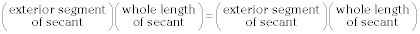##### Geometry: 1,001 Practice Problems For Dummies (+ Free Online Practice)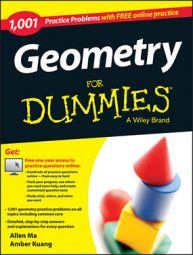To solve geometry problems about circles, you will need to know the following circle theorems involving tangents, secants, and chords. These theorems can be used to find information about angles, intercepted arcs, and length of segments of a circle. In addition, you find the standard and general form of a circle, the formulas for area and circumference, and the area of a sector of a circle.

## Circle formulas

• The circumference of a circle equals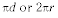• The area of a circle equals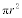• The area of a sector equals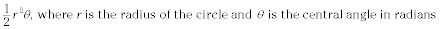•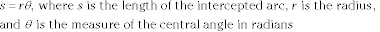• Standard form of a circle: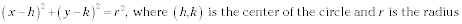• General form of a circle: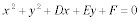## Circle theorems involving angles

• The central angle equals the intercepted arc.

• An inscribed angle equals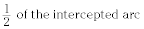• The interior vertical angles formed by two intersecting chords equal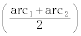• An exterior angle equals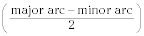• A line tangent to a circle is perpendicular to the radius drawn to the point of tangency.

## Circle theorems involving lengths of segments

• When a tangent and secant are drawn from the same exterior point,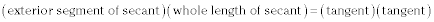• When two secants are drawn from the same exterior point,